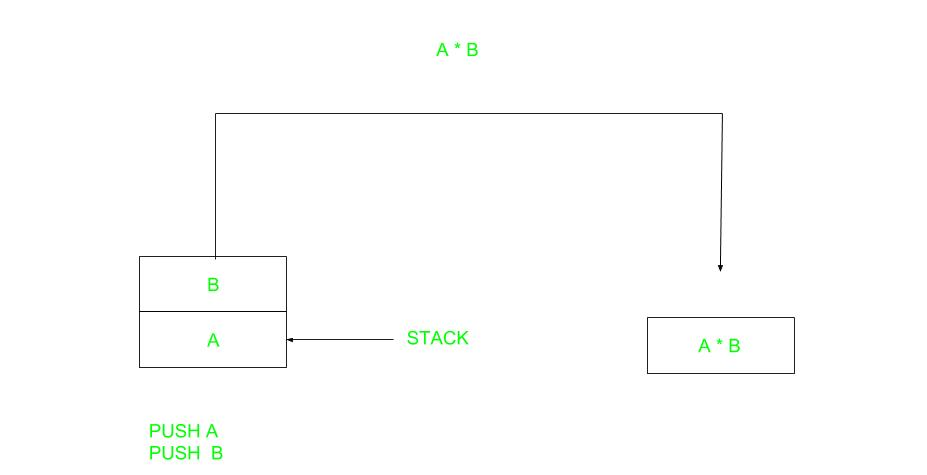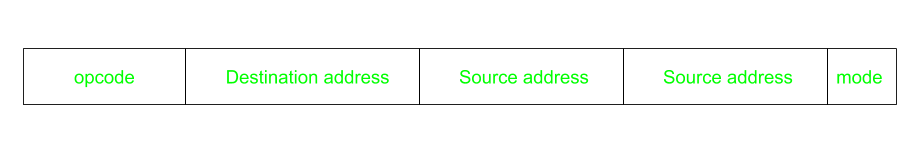# Computer Organization | Instruction Formats (Zero, One, Two and Three Address Instruction)

Computer perform task on the basis of instruction provided. An instruction in computer comprises of groups called fields. These field contains different information as for computers every thing is in 0 and 1 so each field has different significance on the basis of which a CPU decide what to perform. The most common fields are:

• Operation field which specifies the operation to be performed like addition.
• Address field which contain the location of operand, i.e., register or memory location.
• Mode field which specifies how operand is to be founded.

An instruction is of various length depending upon the number of addresses it contain. Generally CPU organization are of three types on the basis of number of address fields:

1. Single Accumulator organization
2. General register organization
3. Stack organization

In first organization operation is done involving a special register called accumulator. In second on multiple registers are used for the computation purpose. In third organization the work on stack basis operation due to which it does not contain any address field. It is not necessary that only a single organization is applied a blend of various organization is mostly what we see generally.

On the basis of number of address, instruction are classified as:

Note that we will use X = (A+B)*(C+D) expression to showcase the procedure.A stack based computer do not use address field in instruction.To evaluate a expression first it is converted to revere Polish Notation i.e. Post fix Notation.

```Expression: X = (A+B)*(C+D)
Postfixed : X = AB+CD+*
TOP means top of stack
M[X] is any memory location```
 PUSH A TOP = A PUSH B TOP = B ADD TOP = A+B PUSH C TOP = C PUSH D TOP = D ADD TOP = C+D MUL TOP = (C+D)*(A+B) POP X M[X] = TOP
This use a implied ACCUMULATOR register for data manipulation.One operand is in accumulator and other is in register or memory location.Implied means that the CPU already know that one operand is in accumulator so there is no need to specify it.```Expression: X = (A+B)*(C+D)
AC is accumulator
M[] is any memory location
M[T] is temporary location```
 LOAD A AC = M[A] ADD B AC = AC + M[B] STORE T M[T] = AC LOAD C AC = M[C] ADD D AC = AC + M[D] MUL T AC = AC * M[T] STORE X M[X] = AC
This is common in commercial computers.Here two address can be specified in the instruction.Unlike earlier in one address instruction the result was stored in accumulator here result cab be stored at different location rather than just accumulator, but require more number of bit to represent address.Here destination address can also contain operand.

```Expression: X = (A+B)*(C+D)
R1, R2 are registers
M[] is any memory location```
 MOV R1, A R1 = M[A] ADD R1, B R1 = R1 + M[B] MOV R2, C R2 = C ADD R2, D R2 = R2 + D MUL R1, R2 R1 = R1 * R2 MOV X, R1 M[X] = R1
This has three address field to specify a register or a memory location. Program created are much short in size but number of bits per instruction increase. These instructions make creation of program much easier but it does not mean that program will run much faster because now instruction only contain more information but each micro operation (changing content of register, loading address in address bus etc.) will be performed in one cycle only.```Expression: X = (A+B)*(C+D)
R1, R2 are registers
M[] is any memory location```
 ADD R1, A, B R1 = M[A] + M[B] ADD R2, C, D R2 = M[C] + M[D] MUL X, R1, R2 M[X] = R1 * R2

Attention reader! Don’t stop learning now. Get hold of all the important CS Theory concepts for SDE interviews with the CS Theory Course at a student-friendly price and become industry ready.

My Personal Notes arrow_drop_upCheck out this Author's contributed articles.

If you like GeeksforGeeks and would like to contribute, you can also write an article using contribute.geeksforgeeks.org or mail your article to contribute@geeksforgeeks.org. See your article appearing on the GeeksforGeeks main page and help other Geeks.

Please Improve this article if you find anything incorrect by clicking on the "Improve Article" button below.